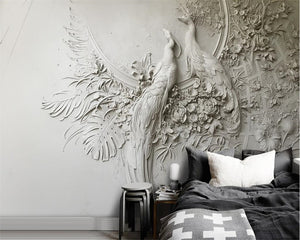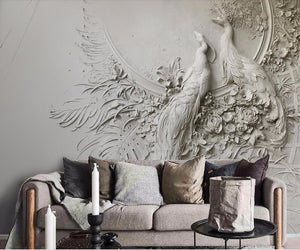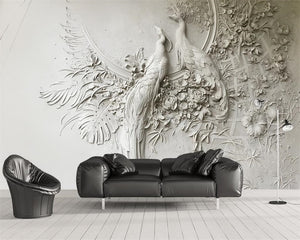# 3D Embossed Peacock Wall Paper

Regular price
\$44.99
Sale price
\$44.99

3D imaging and embossed 'Peacocks' wall paper art.

Suitable for all areas: office, foyer, entry, living room, bedroom, dining room, media room. Mixed styles of classic, elegant sophisticated, modern, art deco and more.

Price is per meter square. (per 1 Quantity)

Quantity 1 : 1 square meter = 140cm(W) x 70cm(H) (4'7" x 2'4") ft
Quantity 2 : 2 square meter = 200cm(W) x 100cm(H) (6'7" x 3'3") ft
Quantity 3 : 3 square meter = 220cm(W) x 140cm(H) (7'3" x 4'7") ft
Quantity 4 : 4 square meter = 250cm(W) x 160cm(H) (8'2" x 5'3") ft
Quantity 5 : 5 square meter = 280cm(W) x 180cm(H) (9'2" x 5'11") ft
Quantity 6 : 6 square meter = 300cm(W) x 200cm(H) (9'10" x 6'7") ft
Quantity 7 : 7 square meter = 330cm(W) x 210cm(H) (10'10" x 6'11") ft
Quantity 8 : 8 square meter = 360cm(W) x 230cm(H) (11'10" x 7'6") ft
Quantity 9 : 9 square meter = 380cm(W) x 240cm(H) (12'5" x 7'10") ft
Quantity 10 : 10 square meter = 400cm(W) x 250cm(H) (13'1" x 8'2") ft
Quantity 11 : 11 square meter = 420cm(W) x 260cm(H) (13'9'' x 8'6'') ft
Quantity 12 : 12 square meter = 440cm(W) x 270cm(H) (14'5" x 8'10") ft

Width x height = square meters (square feet)

For example if you need wall paper to fit wall size: Width 2.8m  Height 1.7m

2.8m x 1.7m = 4.76m2 round up, you will need to order Quantity: 5

Adhesive not included.

Covering a mixture of different tastes and styles - we like that!